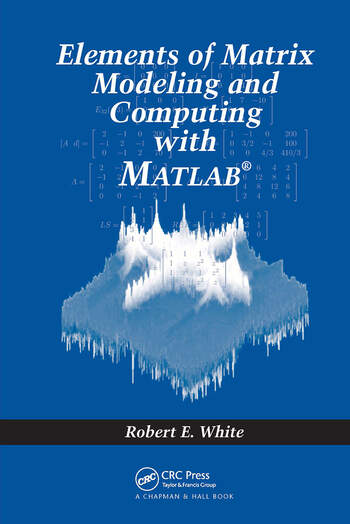Elements of Matrix Modeling and Computing with MATLAB

1st Edition

Robert E. White

Chapman and Hall/CRC
Published September 12, 2006
Textbook - 402 Pages - 116 B/W Illustrations
ISBN 9781584886273 - CAT# C627

For Instructors Request Inspection Copy

USD\$115.00

FREE Standard Shipping!

Preview

Summary

As discrete models and computing have become more common, there is a need to study matrix computation and numerical linear algebra. Encompassing a diverse mathematical core, Elements of Matrix Modeling and Computing with MATLAB examines a variety of applications and their modeling processes, showing you how to develop matrix models and solve algebraic systems. Emphasizing practical skills, it creates a bridge from problems with two and three variables to more realistic problems that have additional variables.

Elements of Matrix Modeling and Computing with MATLAB focuses on seven basic applications: circuits, trusses, mixing tanks, heat conduction, data modeling, motion of a mass, and image filters. These applications are developed from very simple to more complex models. To explain the processes, the book explores numerous topics in linear algebra, including complex numbers and functions, matrices, algebraic systems, curve fitting, elements of linear differential equations, transform methods, and tools of computation. For example, the author uses linearly independent vectors and subspaces to explain over- and under-determined systems, eigenvalues and eigenvectors to solve initial value problems, and discrete Fourier transforms to perform image filtering in the frequency domain. Although the primary focus is to cultivate calculation skills by hand, most chapters also include MATLAB to help with more complicated calculations.

Instructors

We provide complimentary e-inspection copies of primary textbooks to instructors considering our books for course adoption.